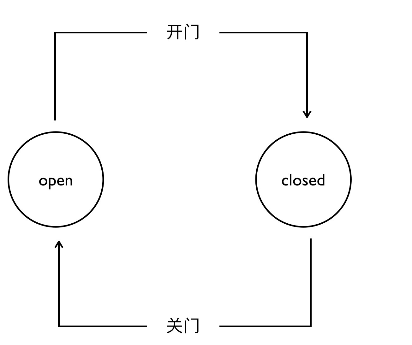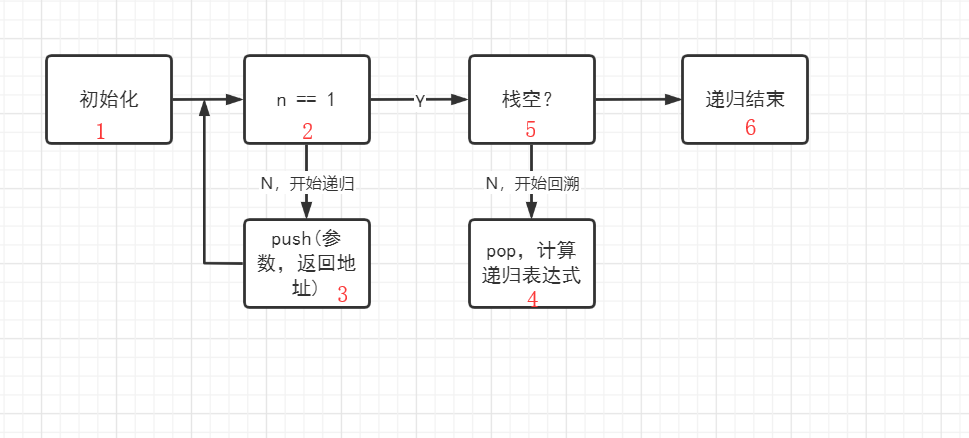### 1. 递归的简介

long long factorial(int n) {
if(n == 1) return 1; // 递归基(出口)
return n * factorial(n - 1);
}

### 3.常见的递归算法

// 二分查找
int sum(int A[], int low, int high)
{
return (low == high) ? A[low] : sum(A, low, (low + high) >> 1) + sum(A, ((low + high) >> 1) + 1, high);
}

// 递归求数组和
int sum(int A[], int n)
{
return (n < 1) ? 0 : A[n - 1] + sum(A, n-1);
}

• 将非尾递归函数变成尾递归函数(可能部分语言不支持)

• 将递归的表达式(即自顶向下)转化为递推表达式(自底向上)

• 使用状态机等方法模拟递归从而消除递归

### 4.状态机的概念### 5.使用状态机消除递归

• 递归过程(自顶向下):如果当前状态不满足递归出口条件，则不断的递归过程，将当前的状态压入堆栈中，直到满足递归出口的条件，停止递归。

• 回溯过程(自底向上)：当递归树上的一分支的递归状态结束之后，不断的进行回溯将栈中保存的内容pop出栈，然后计算递归表达式，直到栈空为止，返回最后的计算结果。#include <iostream>
#include <stack>
​
struct Data {
int num; // 方法的参数
};
​
std::stack<Data> my_stk;
​
int execute_factorial(int n) {
int state = 1; // 初始状态为1
int res = 1;
while(state != 6) { // 当状态为6时结束递归
switch(state) {
case 1: // 递归初始化状态
state = 2;
break;
case 2: // 判断是否到达递归出口
if(n <= 1) {
res = 1;
state = 4; // 递归过程完成，进入回溯状态
} else
state = 3; // 继续递归过程
​
break;
case 3: // 递归入栈
my_stk.push({n, 0});
--n; // 每递归一次n减1
state = 2;
break;
case 4: // 栈是否为空
if(my_stk.empty())
state = 6;
else
state = 5;
break;
case 5: // 回溯过程
Data tmp =my_stk.top();
my_stk.pop();
res *= tmp.num;
state = 4;
break;
}
}
return res;
}
​
int main()
{
std::cout << execute_factorial(5) << std::endl;
return 0;
}8-毛俊杰 前源码公社社长兼任编程俱乐部部长，ICPC竞赛铜牌退役狗，专攻算法和机器学习，博客：https://blog.dalao.icu